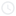6 December, 20203 min read

# Graphical Representation of Javascript Closure

In the following example, every time the function add gets executed, a brand new execution context is created and after the execution of the function is completed, the state is deleted without being able to ever access state from previous functions run again.

123456789
`function add(inputNumber) {`
`    const result = inputNumber + 10;`
`    return result;`
`}`

`const number1 = add(3);`
`const number2 = add(6);`
`console.log(number1);`
`console.log(number2);`

However, it might be useful to access state from previous runs of the function later on in our programs, for example in case we need to rely on previous execution state of the function and on previous state.

The following code will do the sum of numbers by storing the result in the variable sum, which is stored in Global Memory as a “backpack”. Will defines in his course a “backpack” as being all the surrounding data that the function brings with it after its execution into the Global Memory.

123456789101112131415
`function sum() {`
`    let sum = 0;`
`    function add(num) {`
`        sum+= num;`
`         return sum;`
`   }`

`    return add;`
`}`

` const addNumber = sum();`
` const number1 = addNumber(3);`
` const number2 = addNumber(6);`
` console.log(number1);`
` console.log(number2);`

First of all, we need to create a new React app:

1234
`npx create-react-app recoil-example`
`cd recoil-example`
`yarn`
`yarn start`

So what exactly happens when we run this code? I will explain all the steps that happen during code execution.I will start executing the code line by line and I will describe what happens during code execution with the help of the memory diagram and I will refer to (step X) when addressing the memory diagram .

At line 1 the function sum is defined in the Global Memory (step 1), not knowing at this moment yet what will be the result of executing the function sum. The execution of the program jumps to line 11 where the constant addNumber is defined. In the Global Memory (step 2) addNumber is uninitialised for now until the execution of sum is finished.

A brand new execution context is created (step 3) and the execution of sum starts. In the Local Memory a new variable sum is defined which is initialised with 0 (line 2). Then the function add is defined and it is returned (line 8). The constant addNumber is now initialised with the function add that was born by executing the function sum, having the variable sum initialised with 0 in the variable `[[scope]]` stored in Global Memory as a “backpack” to the addNumber constant (step 4).

At line 12 a constant number1 is defined, which remains uninitialised for now (step 5), but will be the result of executing the method addNumber with parameter 3 (step 6). When the function addNumber is executed, a brand new execution context is created. The sum variable is searched in the Local Memory, it is not found there, then it is found in the [[scope]] of addNumber which is its “backpack”. sum is updated to 3 in the “backpack” and number1 is initialised with 3 (step 7).

At line 13, the constant number2 is defined (step 8), which remains uninitialised until the execution of addNumber (step 9) finishes. A brand new execution context is created, where num is initialised to 6 and sum is returned with the value 9 into the variable number2 and the value in the “backpack” of addNumber is updated to 9 (step 10).

At line 14 and 15 values 3 and 9 are printed into the console.

Please let me know in the comments section below if you liked this article and if you find it helpful to see a a graphical representation of closure.

Topics
EngineeringJavaScriptJavaScriptClosure